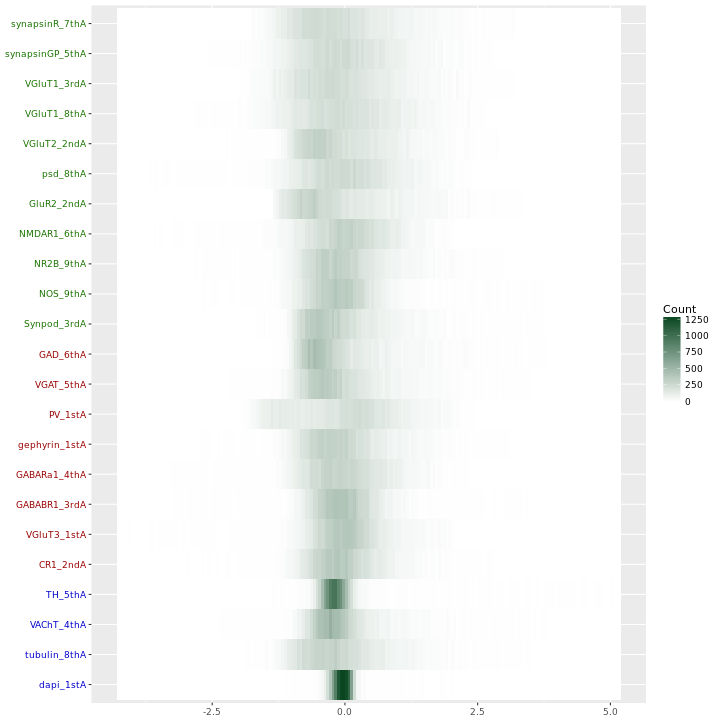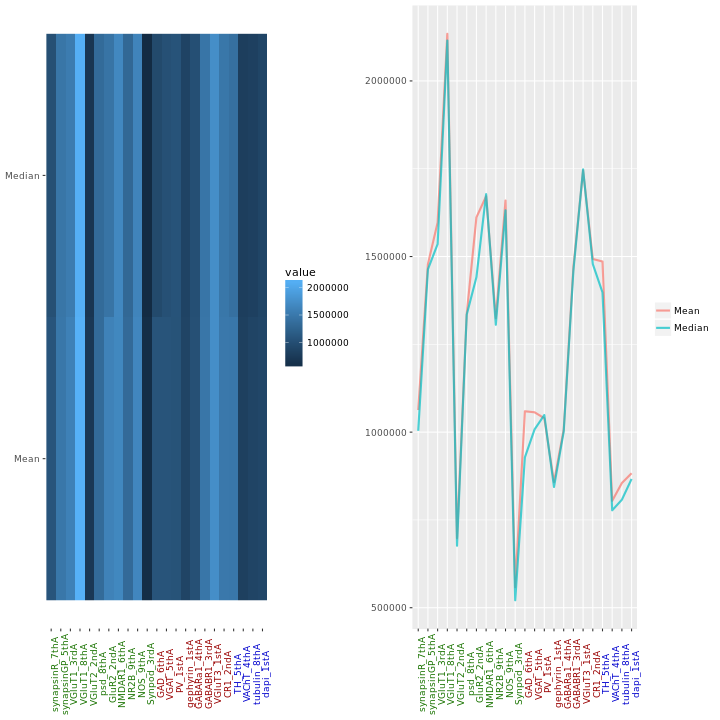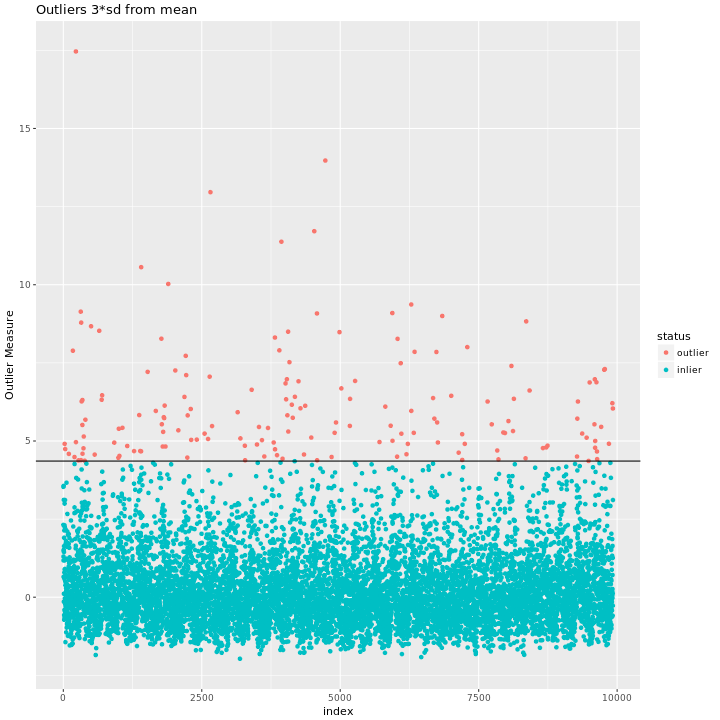# 1 Synaptome Statistics: Notes

Putative synapse locations have been detected in K15 with Forrest’s synapse detection algorithm and in W with Anish’s synapse detection algorithm. For each feature channel (Synapsin, VGlut, psd95, etc.) an 11x11x11 cube is extracted around each each putative synapse location and the voxel values are summed, creating a feature vector of length (number of channels). This gives us an $$n \times d$$ matrix, where the $$n$$ rows correspond to putative synapses and the $$d$$ columns correspond to the summed immunoflorescence in each channel.

## 1.1 Clustering

We have implemented our own Hierarchical Mclust function by augmenting Mclust. In the course of exploring we used the full suite of models available in mclustModelNames p. 88

After looking through the BIC plots of each of the 11 models for each node of the tree it seemed best to use the unconstrained model “VVV” = ellipsoidal, varying volume, shape, and orientation.

## 1.2 MEDA Run on Z-scored data

### 1.2.1 1-d Heatmap### 1.2.2 Location plots### 1.2.3 Outliers as given by randomForest